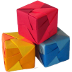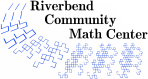# Sonobe Origami Polyhedra – Links

### Origami as a Field of Mathematics

• Several books have been written summarizing the presentations made at a series of international Origami in Science, Mathematics, and Engineering (OSME) Conferences. For a visual overview of the field of mathematical origami see Erik Demaine's photos from the 3rd International Meeting of OSME. Searching for OSME online will lead to many books, abstract, and photographs.
• Liz Newton's excellent article, The Power of Origami explains more details about the history of mathematical origami, Huzita's origami axioms, origami constructions, and computational folding.
• Eric Weisstein's World of Mathematics gives an overview of some results in mathematical origami.
• Tom Hull's mathematical origami page is the most comprehensive source for information on mathematical origami that I have seen. The notes from his Combinatorial Geometry class survey many approaches to mathematical origami. Fields of mathematics invoked in Tom's course are convex polyhedral geometry (graph theory), Huzita's origami geometry axioms, and combinatorial modelling of paper folding (flat folding). Tom has also published a number of mathematical origami papers. To get a feel for the scope of the field of mathematical orgiami, you can browse through Tom Hull's Origami Math Bibliography and the proceedings of the 3rd International Meeting of Origami Science, Math, and Education.
• Robert Lang's web page shows his many amazing origami designs and also discusses mathematical origami. You can also download his TreeMaker algorithm which can be used to design new origami bases.
• Helena Verrill considers several mathematical origami questions on her web page. She also has a paper on Origami Tessellations.
• Koshiro shows how to use basic rules of origami construction to divide a square paper into arbitrarily many equal strips. This discussion includes proofs of several of Haga's theorems.
• Erik Demaine's Folding and Unfolding Page gives an overview of folding and unfolding problems with links to mathematical details and papers. Erik's primary interest is in finding algorithms that characterize foldability in different objects. The objects being folded range from paper, to robot arms, to proteins. See also Joe O'Rourke and Komei Fukada's page on unfolding convex polytopes.
• David Eppstein, the founder of the Geometry Junkyard, has posted a mathematical bull session on the Margulis Napkin Problem. This problem asks for a proof that it is impossible to fold a unit square to form a flat shape whose perimeter is greater than 4. In the course of the discussion, the participants realize that the problem is wrong as stated -- there is a way to fold a unit square to form a flat shape whose perimeter is greater than 4, and this turns out to be the key to creating many origami animals. Helena Verrill gives a clearer presentation of how to construct an origami counter-example.
• David Eppstein's site hosted another mathematical bull session on the Teabag Problem. This problem asks for the maximum volume a teabag can hold. A number of mathematicians contributed to the original discussion.
• Roger Alperin defines and proves theorems about Origami numbers, numbers that are constructible by origami folds.
Riverbend Community Math Center
hello@riverbendmath.org
http://riverbendmath.org
(574) 339-9111This work placed into the public domain by the Riverbend Community Math Center.